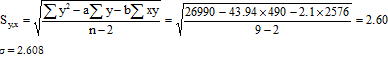# Answered! Use trend projection with regression to forecast sales for weeks 10-13. What are the error measures (CFE, MSE…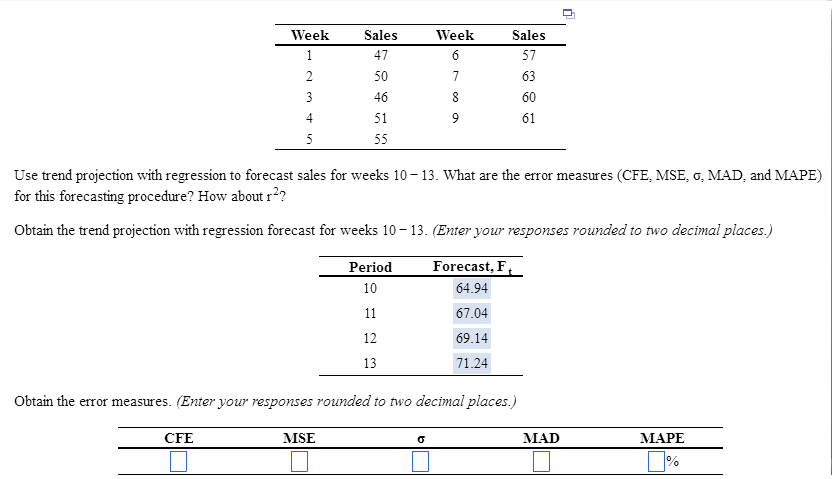Use trend projection with regression to forecast sales for weeks 10-13. What are the error measures (CFE, MSE, sigma MAD, and MAPE) for this forecasting procedure? How about r^2? Obtain the trend projection with regression forecast for weeks 10-13. (Enter your responses rounded to two decimal places.) Obtain the error measures. (Enter your responses rounded to two decimal places.)

Trend Projection Method

Least square method is applied to determine to a linear trend line which is given by following equation:

Don't use plagiarized sources. Get Your Custom Essay on
Answered! Use trend projection with regression to forecast sales for weeks 10-13. What are the error measures (CFE, MSE…
GET AN ESSAY WRITTEN FOR YOU FROM AS LOW AS \$13/PAGE

Y = a + bx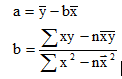Where,

y = Sales

x = quarter number

Y = demand computed using regression equation

a = y-axis intercept

b = slope of the line

 x y x2 x*y y2 1 47 1 47 2209 2 50 4 100 2500 3 46 9 138 2116 4 51 16 204 2601 5 55 25 275 3025 6 57 36 342 3249 7 63 49 441 3969 8 60 64 480 3600 9 61 81 549 3721 45 490 285 2576 26990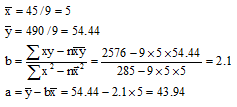The regression line is given by formula:

Y = a + bx = 43.94 + 2.1x

Forecast value for the next months are:

 x Y = 43.9 + 2.1x 10 Y = 43.9 + 2.1*10 = 64.94 11 Y = 43.9 + 2.1*11 = 67.04 12 Y = 43.9 + 2.1*12 = 69.14 13 Y = 43.9 + 2.1*13 = 71.24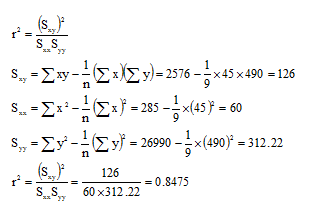Calculating Errors:

 Forecast Error Absolute Sum of % E x y yc=43.9+2.1x E = y-yc |E| CSE E2 100|E|/y 1 47 46.0444 0.9556 0.9556 0.9556 0.9131 2.0331 2 50 48.1444 1.8556 1.8556 2.8111 3.4431 3.7111 3 46 50.2444 -4.2444 4.2444 -1.4333 18.0153 9.2271 4 51 52.3444 -1.3444 1.3444 -2.7778 1.8075 2.6362 5 55 54.4444 0.5556 0.5556 -2.2222 0.3086 1.0101 6 57 56.5444 0.4556 0.4556 -1.7667 0.2075 0.7992 7 63 58.6444 4.3556 4.3556 2.5889 18.9709 6.9136 8 60 60.7444 -0.7444 0.7444 1.8444 0.5542 1.2407 9 61 62.8444 -1.8444 1.8444 0.0000 3.4020 3.0237 Total 0.0000 16.3556 0.0000 47.6222 30.5947

Forecast sales for each month are calculated by formula: yc = 43.94 + 2.1x

Forecast Error (E) for each month = E = y – yc

Cumulative forecast error= CFE = ∑( y – yc ) = 0

Mean Squared Error (MSE) = [∑( y – yc )2]/n = [∑E2]/9 = 47.622/9 =5.2913

MSE = 5.2913

Mean Absolute Deviation = [∑|y – yc|]/n = [∑|E|]/9 = 16.3556/9 = 1.8172

Mean Absolute Percent Error = MAPE = (1/n) x (∑100|E|/y) = (1/9)x(30.5947)=3.34

Standard Error of Estimate (Sy,x ) = Standard deviation of regression (σ)